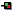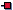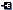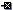## More Storage Containers

We have examined in passing the use of vectors (`java.util.vector`) to store data. This section is going to discuss Java storage containers in a bit more detail. First we will look at how arrays can be used to provide some more functionality within Java.

### More Java Arrays

Before we begin, you should ensure that you are familiar with the standard Java arrays. To check that you are familiar I would ask you to solve this very short problem yourself: Write a Java application that reads in a number of strings from the command line and provides a histogram of the numbers of letters in the words passed e.g. if the program was executed as: java ArrayApp This is a test string it would return 1 word with 1 letter, 1 words with 2 letters, 2 words with 4 letters etc. The solution to this problem is at the bottom of the page.

#### Sorting a Java Array

We have seen how to sort an array in C++ using STL. In Java, there is a helper class for working with arrays called`java.util.Arrays` that provides functionality such as sorting and efficient searching to our arrays. For example, if we wish to sort an array of Strings, we could use:

```import java.util.Arrays;

public class SortArrays {

public static void main(String[] args) {
Arrays.sort(args);
for(int i=0; i<args.length; i++)
{
System.out.println(args[i]);
}
}
}
```

Which will execute as:

```C:\temp\java SortArrays Dog Bird Cat Apple
Apple
Bird
Cat
Dog
```

It is therefore quite straightforward to sort Strings and indeed intfloat etc. arrays. It gets more complicated when we wish to sort objects of our own type. You will remember that in C++ we had to override the operators that operated on the data type (e.g. equals, less than, more than). In Java we have to do pretty much the same thing, but since we have no operator overloading we must use interfaces, the`java.util.Comparator` interface in particular.

Suppose we have a straightforward`Student` class, where we have exposed the private name and id states through the`getName()` and`getID()` accessor methods:

 1234567891011121314151617181920 `package ee402;public class Student extends Object{        private String name;        private int id;                public Student(String name, int id){         this.name = name;         this.id = id;        }                public void display(){         System.out.println("A student with name: " + name          + " and id " + id);        }        public String getName() { return name; }        public int getID() { return id; }}`

We can provide comparisons of the states of this class by implementing the`Comparator` interface as follows:

 1234567891011 `package ee402;import java.util.Comparator;public class StudentIDComparator implements Comparator {        public int compare(Student a, Student b) {         if (a.getID() < b.getID()) return -1;         else if (a.getID() > b.getID()) return 1;         else return 0;        }}`

Where the comparison returns -1 in the case where the left object is less than the right object, +1 in the opposite case and 0 when the two objects are numerically identical

In the case of comparing two strings it is fairly straightforward as we can just use the`String``compareTo()` method which performs the comparison as we require.

 12345678910 `package ee402;import java.util.Comparator;public class StudentNameComparator implements Comparator {        public int compare(Student a, Student b) {         return a.getName().compareTo(b.getName());        }}`

Finally, we write the application code to use our comparators with our data type:

 12345678910111213141516171819202122232425262728293031323334 `package ee402;import java.util.Arrays;import java.util.Comparator; //Comparator Interfacepublic class SortingObjects {                public static void main(String[] args) {                        Comparator byName = new StudentNameComparator();                Comparator byID = new StudentIDComparator();                                Student[] students = new Student[]{                        new Student("Derek", 1234),                        new Student("Sarah", 1238),                        new Student("Mick", 1237),                        new Student("Adam", 1233),                        new Student("Jane", 1231)};                                                // Sort by Name                Arrays.sort(students, byName);                System.out.println("The students sorted by name:");                for (int i=0; i

On execution this will give the output:

```The students sorted by name:
A student with name: Adam and id 1233
A student with name: Derek and id 1234
A student with name: Jane and id 1231
A student with name: Mick and id 1237
A student with name: Sarah and id 1238

The students sorted by id:
A student with name: Jane and id 1231
A student with name: Adam and id 1233
A student with name: Derek and id 1234
A student with name: Mick and id 1237
A student with name: Sarah and id 1238
```

As you can imagine it is possible to make quite advanced comparisons between objects of the class that you define.

### Java Generics

I have given the example before of creating a`Vector` of objects, and as the`Object` class is the parent of all classes in Java, we are able to store any object of a class in such a`Vector`. Now, this is a useful feature of the language; however, it does not provide us with compile-time safety with our code. For example, we could accidentally try to convert a`String` object into a`Fish` object, but this would only be determined at run time, which will also likely result in a`ClassCastException`.

Generics in Java provides us with a better way. We can create a typed container of elements. For example, we could create a`List` of students using:

```List<Student> studentList = new ArrayList<Student>();
```

As opposed to the old syntax:

```List studentList = new ArrayList();
```

Now, this line of code will be the same for either version:

```studentList.add(new Student("Derek", 1234));
```

However, one advantage of the generics version is that if we try to add an incorrect value to the`ArrayList` we will get a compile time error; so, for example if we incorrectly added a`String` object to our `studentList` using:

```studentList.add(new String("Test"));
```

it will be allowed for the old syntax, but will give a compile-time error with the generics version. In addition the behaviour is different when we wish to operate on the data that we take off the list. With the old syntax version we would have to write:

```	List studentList = new ArrayList();
Student s = (Student) studentList.get(0);
System.out.println(s.getName());
```

Whereas, with the generics version we would have:

```	List<Student> studentList = new ArrayList<Student>();
Student s = studentList.get(0);
System.out.println(s.getName());
```

So, we no longer have to cast the object of the`Object` class to a`Student` object; rather, the`List` stores a list of`Student` objects. Again, the big advantage with the generics version is that the errors will be detected at compile time, which is much less expensive than detecting errors at run time.

With the use of generics also comes an enhanced for loop. For example, if we wished to iterate through a`Vector` of students we could use the three following syntax examples:

 1234567891011121314151617181920212223242526272829303132333435363738394041424344 `package ee402;import java.util.*;public class GenericsTest {        public static void main(String args[])        {         // Populate a Vector using an array of Students         Student[] studentArray = new Student[]{         new Student("Derek", 1234),         new Student("Tom", 1235),         new Student("Jane", 1236),         new Student("Jill", 1237)         };                  Vector students = new Vector(10);         students.addAll(Arrays.asList(studentArray));                  // Iterate the usual way         System.out.println("The Usual Way:");         for (int i=0; i e = students.elements();         while(e.hasMoreElements())         {         Student tempStudent = e.nextElement();         System.out.print(tempStudent.getName() + ", ");         }                  // Using the "enhanced for"         System.out.println("\nUsing the enhanced For:");         for(Student tempStudent : students)         {         System.out.print(tempStudent.getName() + ", ");         }        }}`

This results in the output:

```The Usual Way:
Derek, Tom, Jane, Jill,
Using an Enumeration:
Derek, Tom, Jane, Jill,
Using the enhanced For:
Derek, Tom, Jane, Jill,
```

which is exactly the same for all three cases.

These notes are copyright Dr. Derek Molloy, School of Electronic Engineering, Dublin City University, Ireland 2013-present. Please contact him directly before reproducing any of the content in any way.
ċ
Derek Molloy,
14 Dec 2013, 05:53